# Data visualization¶

The object returned by `load()`, a `BaseSignal` instance, has a `plot()` method that is powerful and flexible tools to visualize n-dimensional data. In this chapter, the visualisation of multidimensional data is exemplified with two experimental datasets: an EELS spectrum image and an EDX dataset consisting of a secondary electron emission image stack and a 3D hyperspectrum , both simoultaneously acquired by recording two signals in parallel in a FIB/SEM.

```>>> s = hs.load('YourDataFilenameHere')
>>> s.plot()
```

if the object is single spectrum or an image one window will appear when calling the plot method.

## Multidimensional spectral data¶

If the object is a 1D or 2D spectrum-image (i.e. with 2 or 3 dimensions when including energy) two figures will appear, one containing a plot of the spectrum at the current coordinates and the other an image of the data summed over its spectral dimension if 2D or an image with the spectral dimension in the x-axis if 1D: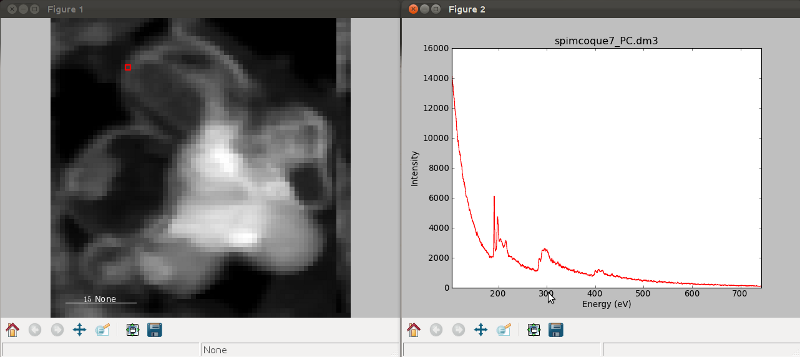Visualisation of a 2D spectrum image.Visualisation of a 1D spectrum image.

To change the current coordinates, click on the pointer (which will be a line or a square depending on the dimensions of the data) and drag it around. It is also possible to move the pointer by using the numpad arrows when numlock is on and the spectrum or navigator figure is selected.When using the numpad arrows the PageUp and PageDown keys change the size of the step.

An extra cursor can be added by pressing the `e` key. Pressing `e` once more will disable the extra cursor: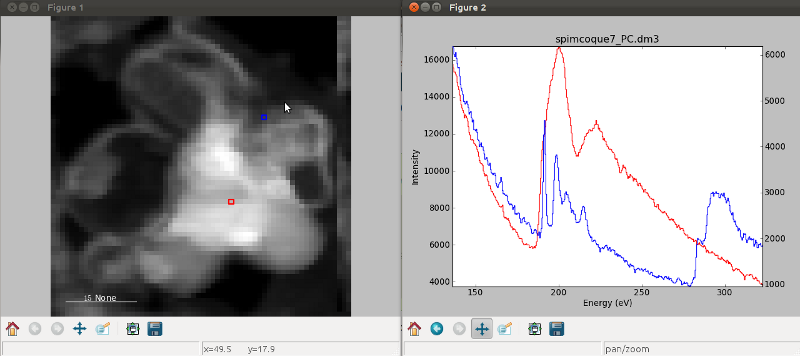Visualisation of a 2D spectrum image using two pointers.

Sometimes the default size of the rectangular cursors used to navigate images can be too small to be dragged or even seen. It is possible to change the size of the cursors by pressing the `+` and `-` keys when the navigator window is selected.

key function
e Switch second pointer on/off
Arrows Change coordinates
PageUp Increase step size
PageDown Decrease step size
`+` Increase pointer size
`-` Decrease pointer size
`h` Launch the contrast adjustment tool (only for Image)

To close all the figures run the following command:

```close('all')
```

Note

This is a matplotlib command. Matplotlib is the library that hyperspy uses to produce the plots. You can learn how to pan/zoom and more in the matplotlib documentation

## Multidimensional image data¶

Equivalently, if the object is a 1D or 2D image stack two figures will appear, one containing a plot of the image at the current coordinates and the other a spectrum or an image obtained by summing over the image dimensions:

The same keys can be used to explore an image stack.

## Customising image plot¶

New in version 0.8.

The image plot can be customised by passing additional arguments when plotting. Colorbar, scalebar and contrast controls are HyperSpy-specific, however matplotlib.imshow arguments are supported as well:

```>>> import scipy
>>> img = hs.signals.Signal2D(scipy.misc.lena())
>>> img.plot(colorbar=True, scalebar=False,
>>>          axes_ticks=True, cmap='RdYlBu_r', saturated_pixels=0)
```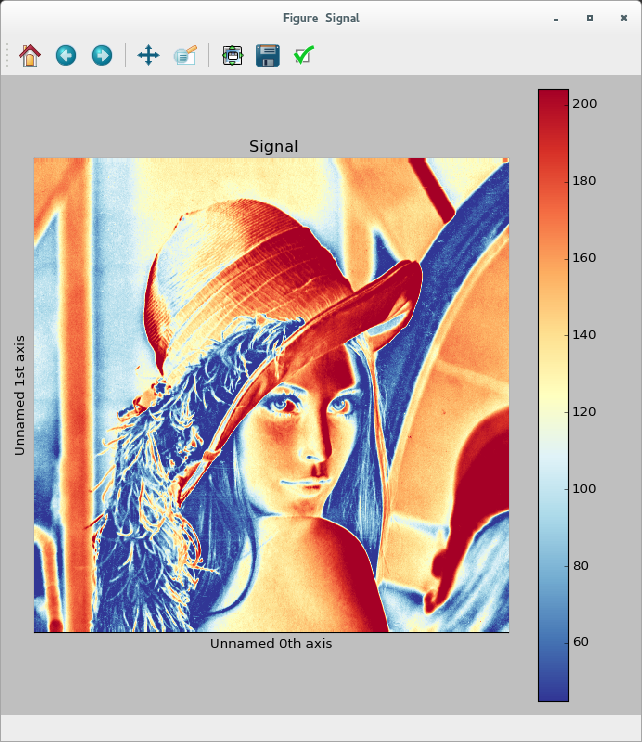Custom colormap and switched off scalebar in an image.

New in version 0.8.1.

When plotting using divergent colormaps, if `centre_colormap` is `True` (default) the constrast is automatically adjusted so that zero corresponds to the center of the colormap (usually white). This can be useful e.g. when displaying images that contain pixels with both positive and negative values.

The following example shows the effect of centering the color map:

```>>> x = np.linspace(-2 * np.pi, 2 * np.pi, 128)
>>> xx, yy = np.meshgrid(x, x)
>>> data1 = np.sin(xx * yy)
>>> data2 = data.copy()
>>> data2[data2 < 0] /= 4
>>> im = hs.signals.Signal2D([data1, data])
>>> hs.plot.plot_images(im, cmap="RdBu", tight_layout=True)
```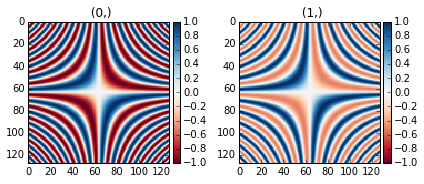Divergent color map with ``Centre colormap `` enabled (default).

The same example with the feature disabled:

```>>> x = np.linspace(-2 * np.pi, 2 * np.pi, 128)
>>> xx, yy = np.meshgrid(x, x)
>>> data1 = np.sin(xx * yy)
>>> data2 = data.copy()
>>> data2[data2 < 0] /= 4
>>> im = hs.signals.Signal2D([data1, data])
>>> hs.plot.plot_images(im, centre_colormap=False, cmap="RdBu", tight_layout=True)
```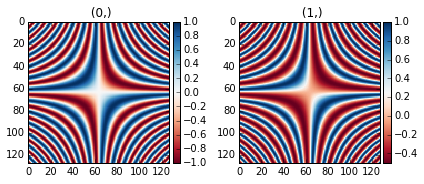Divergent color map with `Centre colormap` disabled.

## Customizing the “navigator”¶

```>>> from urllib import urlretrieve
>>> url = 'http://cook.msm.cam.ac.uk//~hyperspy//EDS_tutorial//'
>>> urlretrieve(url + 'TiFeNi_010.rpl', 'Ni_superalloy_010.rpl')
>>> urlretrieve(url + 'TiFeNi_010.raw', 'TiFeNi_010.raw')
>>> urlretrieve(url + 'TiFeNi_012.rpl', 'TiFeNi_012.rpl')
>>> urlretrieve(url + 'TiFeNi_011.raw', 'TiFeNi_011.raw')
>>> urlretrieve(url + 'image010.tif', 'image010.tif')
>>> urlretrieve(url + 'image011.tif', 'image011.tif')
```

Note

Note

The sample and the data used in this chapter are described in P. Burdet, et al., Acta Materialia, 61, p. 3090-3098 (2013) (see abstract).

Stack of 2D images can be imported as an 3D image and plotted with a slider instead of the 2D navigator as in the previous example.

```>>> img = hs.load('image*.tif', stack=True)
>>> img.plot(navigator='slider')
```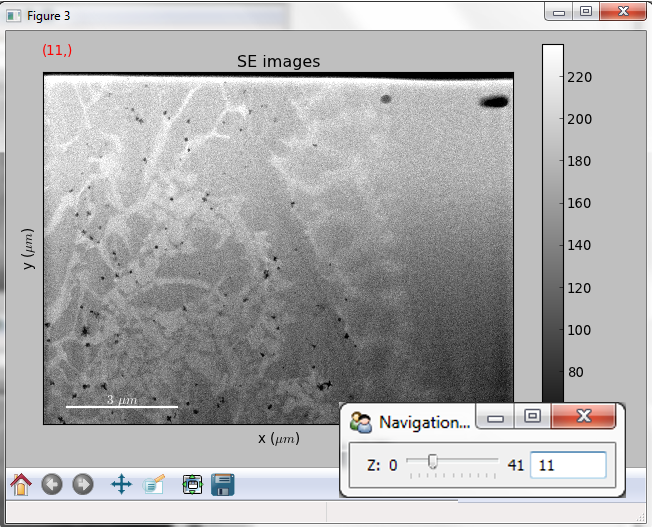Visualisation of a 3D image with a slider.

A stack of 2D spectrum images can be imported as a 3D spectrum image and plotted with sliders.

```>>> s = hs.load('TiFeNi_0*.rpl', stack=True).as_spectrum(0)
>>> s.plot()
```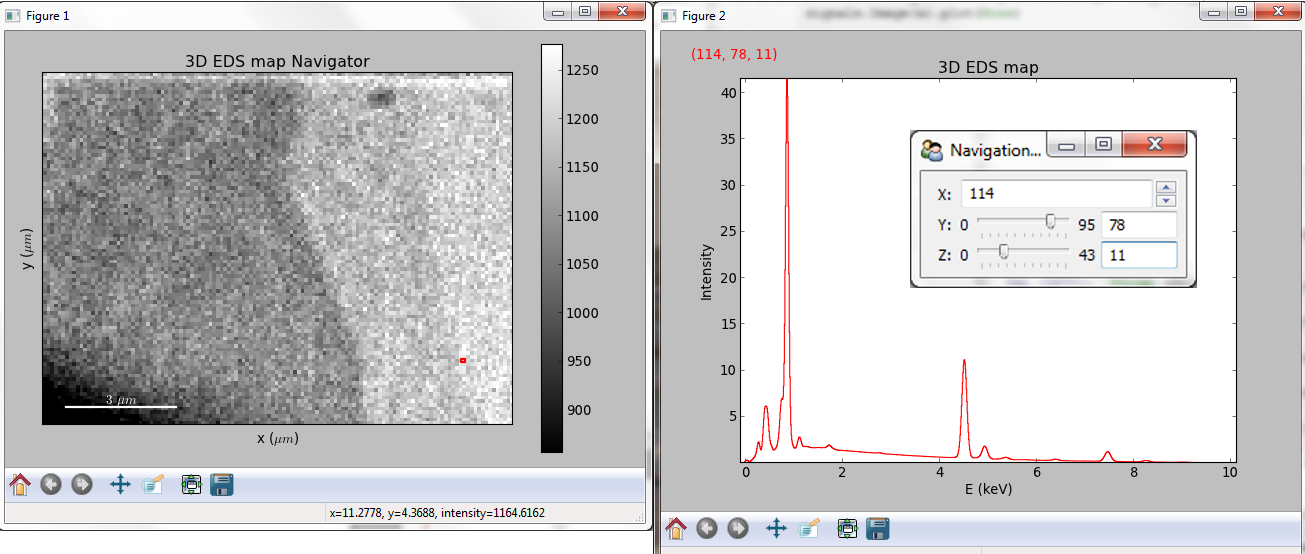Visualisation of a 3D spectrum image with sliders.

If the 3D images has the same spatial dimension as the 3D spectrum image, it can be used as an external signal for the navigator.

```>>> im = hs.load('image*.tif', stack=True)
>>> #Rebin the image
>>> im = im.rebin([dim, dim, dim])
>>> s.plot(navigator=im)
```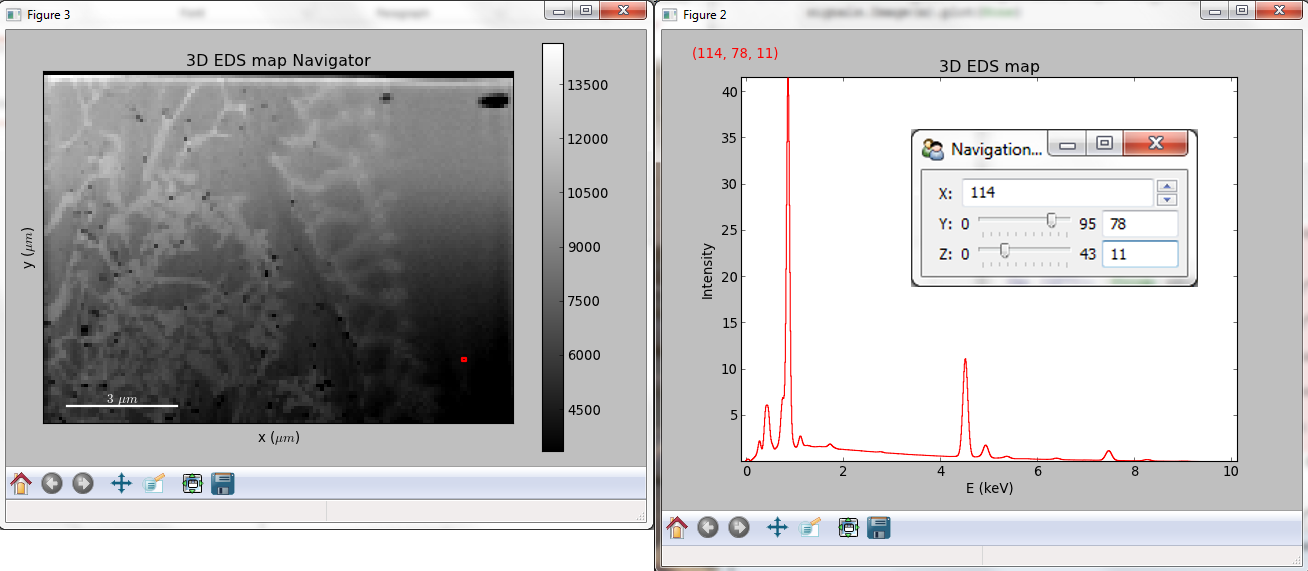Visualisation of a 3D spectrum image. The navigator is an external signal.

The 3D spectrum image can be transformed in a stack of spectral images for an alternative display.

```>>> imgSpec = hs.load('TiFeNi_0*.rpl', stack=True)
>>> imgSpec.plot(navigator='spectrum')
```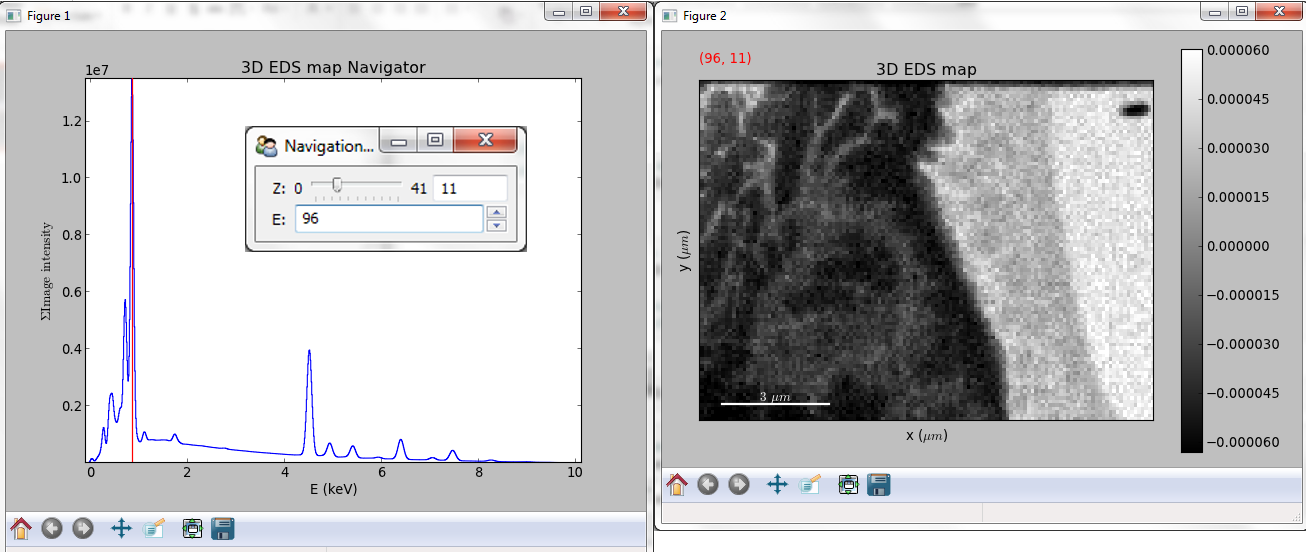Visualisation of a stack of 2D spectral images.

An external signal (e.g. a spectrum) can be used as a navigator, for example the “maximum spectrum” for which each channel is the maximum of all pixels.

```>>> imgSpec = hs.load('TiFeNi_0*.rpl', stack=True)
>>> specMax = imgSpec.max(-1).max(-1).max(-1).as_spectrum(0)
>>> imgSpec.plot(navigator=specMax)
```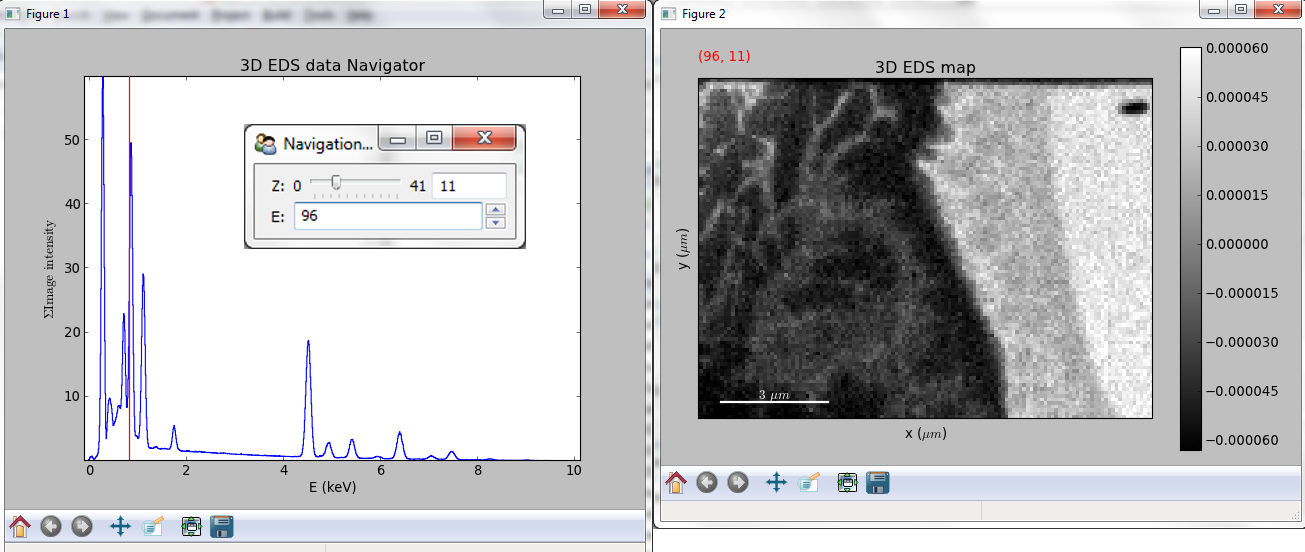Visualisation of a stack of 2D spectral images. The navigator is the “maximum spectrum”.

Lastly, if no navigator is needed, “navigator=None” can be used.

## Using Mayavi to visualize 3D data¶

```>>> from urllib import urlretrieve
>>> url = 'http://cook.msm.cam.ac.uk//~hyperspy//EDS_tutorial//'
>>> urlretrieve(url + 'Ni_La_intensity.hdf5', 'Ni_La_intensity.hdf5')
```

Note

Although HyperSpy does not currently support plotting when signal_dimension is greater than 2, Mayavi can be used for this purpose.

In the following example we also use scikit-image for noise reduction. More details about `get_lines_intensity()` method can be found in EDS lines intensity.

```>>> from mayavi import mlab
>>> mlab.figure()
>>> mlab.contour3d(ni.data, contours=)
>>> mlab.outline(color=(0, 0, 0))
```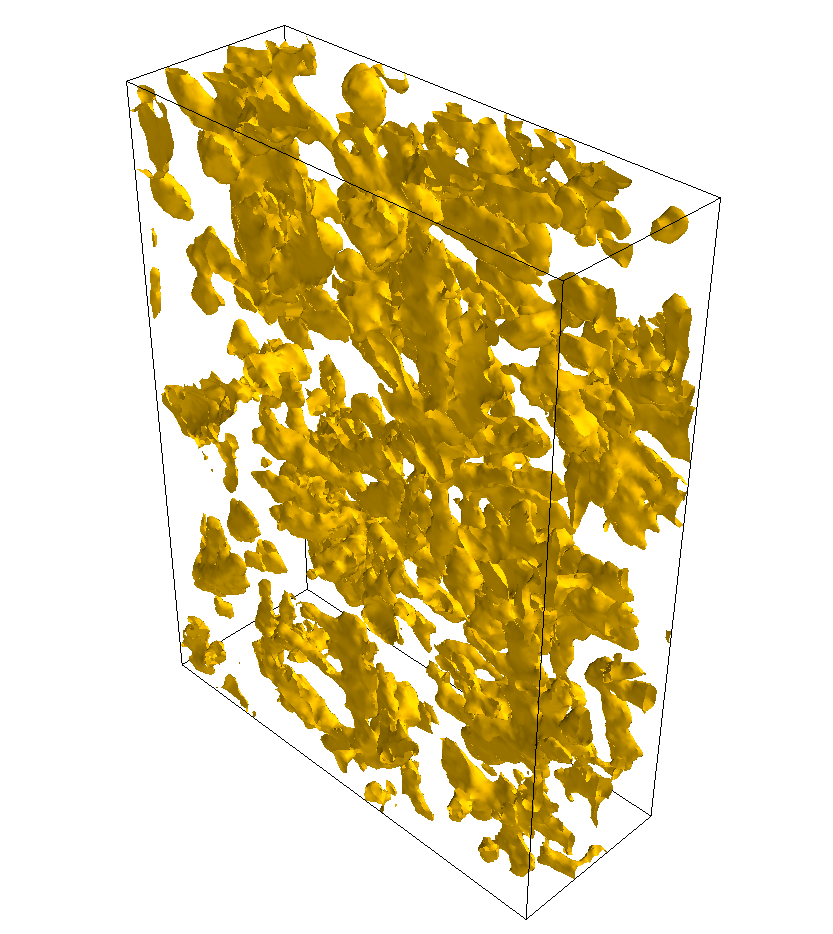Visualisation of isosurfaces with mayavi.

Note

Note

The sample and the data used in this chapter are described in P. Burdet, et al., Ultramicroscopy, 148, p. 158-167 (2015).

## Plotting multiple signals¶

HyperSpy provides three functions to plot multiple signals (spectra, images or other signals): `plot_images()`, `plot_spectra()`, and `plot_signals()` in the `utils.plot` package.

### Plotting several images¶

New in version 0.8.

`plot_images()` is used to plot several images in the same figure. It supports many configurations and has many options available to customize the resulting output. The function returns a list of matplotlib axes, which can be used to further customize the figure. Some examples are given below.

A common usage for `plot_images()` is to view the different slices of a multidimensional image (a hyperimage):

```>>> import scipy
>>> image = hs.signals.Signal2D([scipy.misc.lena()]*6)
>>> angles = hs.signals.Signal(range(10,70,10))
>>> angles.axes_manager.set_signal_dimension(0)
>>> image.map(scipy.ndimage.rotate, angle=angles, reshape=False)
>>> hs.plot.plot_images(image, tight_layout=True)
```

This example is explained in Signal iterator.

By default, `plot_images()` will attempt to auto-label the images based on the Signal titles. The labels (and title) can be customized with the suptitle and label arguments. In this example, the axes labels and the ticks are also disabled with axes_decor:

```>>> import scipy
>>> image = hs.signals.Signal2D([scipy.misc.lena()]*6)
>>> angles = hs.signals.Signal(range(10,70,10))
>>> angles.axes_manager.set_signal_dimension(0)
>>> image.map(scipy.ndimage.rotate, angle=angles, reshape=False)
>>> hs.plot.plot_images(
>>>     image, suptitle='Turning Lena', axes_decor='off',
>>>     label=['Rotation ' + str(angle.data) +
>>>            '\$^\degree\$' for angle in angles], colorbar=None)
```

`plot_images()` can also be used to easily plot a list of Images, comparing different Signals, including RGB images. This example also demonstrates how to wrap labels using labelwrap (for preventing overlap) and using a single colorbar for all the Images, as opposed to multiple individual ones:

```>>> import scipy

>>> # load red channel of raccoon as an image
>>> image0 = hs.signals.Signal2D(scipy.misc.face()[:,:,0])
>>> image0.metadata.General.title = 'Rocky Raccoon - R'

>>> # load lena into 6 hyperimage
>>> image1 = hs.signals.Signal2D([scipy.misc.lena()]*6)
>>> angles = hs.signals.Signal(range(10,70,10))
>>> angles.axes_manager.set_signal_dimension(0)
>>> image1.map(scipy.ndimage.rotate, angle=angles, reshape=False)

>>> # load green channel of raccoon as an image
>>> image2 = hs.signals.Signal2D(scipy.misc.face()[:,:,1])
>>> image2.metadata.General.title = 'Rocky Raccoon - G'

>>> # load rgb image of the raccoon
>>> rgb = hs.signals.Signal1D(scipy.misc.face())
>>> rgb.change_dtype("rgb8")
>>> rgb.metadata.General.title = 'Raccoon - RGB'

>>> images = [image0, image1, image2, rgb]
>>> for im in images:
>>>     ax = im.axes_manager.signal_axes
>>>     ax.name, ax.name = 'x', 'y'
>>>     ax.units, ax.units = 'mm', 'mm'
>>> hs.plot.plot_images(images, tight_layout=True,
>>>                        colorbar='single', labelwrap=20)
```

Data files used in the following example can be downloaded using (These data are described in D. Roussow et al., Nano Lett, 10.1021/acs.nanolett.5b00449 (2015)).

```>>> from urllib import urlretrieve
>>> url = 'http://cook.msm.cam.ac.uk//~hyperspy//EDS_tutorial//'
>>> urlretrieve(url + 'core_shell.hdf5', 'core_shell.hdf5')
```

Another example for this function is plotting EDS line intensities see EDS chapter. One can use the following commands to get a representative figure of the X-ray line intensities of an EDS spectrum image. This example also demonstrates changing the colormap (with cmap), adding scalebars to the plots (with scalebar), and changing the padding between the images. The padding is specified as a dictionary, which is used to call subplots_adjust method of matplotlib (see documentation).

```>>> si_EDS = hs.load("core_shell.hdf5")
>>> im = si_EDS.get_lines_intensity()
>>> hs.plot.plot_images(
>>>     im, tight_layout=True, cmap='RdYlBu_r', axes_decor='off',
>>>     colorbar='single', saturated_pixels=2, scalebar='all',
>>>     scalebar_color='black', suptitle_fontsize=16,
>>>              'right':0.85, 'wspace':0.20, 'hspace':0.10})
```

Note

This padding can also be changed interactively by clicking on the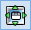button in the GUI (button may be different when using different graphical backends).

### Plotting several spectra¶

`plot_spectra()` is used to plot several spectra in the same figure. It supports different styles, the default being “overlap”. The default style is configurable in preferences.

In the following example we create a list of 9 single spectra (gaussian functions with different sigma values) and plot them in the same figure using `plot_spectra()`. Note that, in this case, the legend labels are taken from the individual spectrum titles. By clicking on the legended line, a spectrum can be toggled on and off.

```>>> s = hs.signals.Signal1D(np.zeros((200)))
>>> s.axes_manager.offset = -10
>>> s.axes_manager.scale = 0.1
>>> m = s.create_model()
>>> g = components.Gaussian()
>>> m.append(g)
>>> gaussians = []
>>> labels = []

>>> for sigma in range(1, 10):
...         g.sigma.value = sigma
...         gs = m.as_signal()
...         gs.metadata.General.title = "sigma=%i" % sigma
...         gaussians.append(gs)
...
>>> hs.plot.plot_spectra(gaussians,legend='auto')
<matplotlib.axes.AxesSubplot object at 0x4c28c90>
```

Another style, “cascade”, can be useful when “overlap” results in a plot that is too cluttered e.g. to visualize changes in EELS fine structure over a line scan. The following example shows how to plot a cascade style figure from a spectrum, and save it in a file:

```>>> import scipy.misc
>>> s = hs.signals.Signal1D(scipy.misc.lena()[100:160:10])
```

The “cascade” style has a padding option. The default value, 1, keeps the individual plots from overlapping. However in most cases a lower padding value can be used, to get tighter plots.

Using the color argument one can assign a color to all the spectra, or specific colors for each spectrum. In the same way, one can also assign the line style and provide the legend labels:

```>>> import scipy.misc
>>> s = hs.signals.Signal1D(scipy.misc.lena()[100:160:10])
>>> color_list = ['red', 'red', 'blue', 'blue', 'red', 'red']
>>> line_style_list = ['-','--','steps','-.',':','-']
>>> line_style=line_style_list,legend='auto')
```

A simple extension of this functionality is to customize the colormap that is used to generate the list of colors. Using a list comprehension, one can generate a list of colors that follows a certain colormap:

```>>> import scipy.misc
>>> fig, axarr = plt.subplots(1,2)
>>> s1 = hs.signals.Signal1D(scipy.misc.lena()[100:160:10])
>>> s2 = hs.signals.Signal1D(scipy.misc.lena()[200:260:10])
>>> hs.plot.plot_spectra(s1,
>>>                         color=[plt.cm.RdBu(i/float(len(s1)-1))
>>>                                for i in range(len(s1))],
>>>                         ax=axarr,
>>>                         fig=fig)
>>> hs.plot.plot_spectra(s2,
>>>                         color=[plt.cm.summer(i/float(len(s1)-1))
>>>                                for i in range(len(s1))],
>>>                         ax=axarr,
>>>                         fig=fig)
>>> fig.canvas.draw()
```

There are also two other styles, “heatmap” and “mosaic”:

```>>> import scipy.misc
>>> s = hs.signals.Signal1D(scipy.misc.lena()[100:160:10])
>>> hs.plot.plot_spectra(s, style='heatmap')
```
```>>> import scipy.misc
>>> s = hs.signals.Signal1D(scipy.misc.lena()[100:120:10])
>>> hs.plot.plot_spectra(s, style='mosaic')
```

For the “heatmap” style, different matplotlib color schemes can be used:

```>>> import matplotlib.cm
>>> import scipy.misc
>>> s = hs.signals.Signal1D(scipy.misc.lena()[100:120:10])
>>> ax = hs.plot.plot_spectra(s, style="heatmap")
>>> ax.images.set_cmap(matplotlib.cm.jet)
```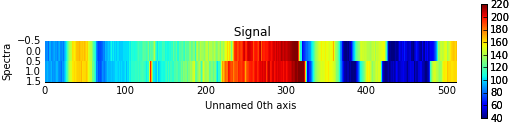Figure generated by `plot_spectra()` using the heatmap style showing how to customise the color map.

Any parameter that can be passed to matplotlib.pyplot.figure can also be used with plot_spectra() to allow further customization (when using the “overlap”, “cascade”, or “mosaic” styles). In the following example, dpi, facecolor, frameon, and num are all parameters that are passed directly to matplotlib.pyplot.figure as keyword arguments:

```>>> import scipy.misc
>>> s = hs.signals.Signal1D(scipy.misc.lena()[100:160:10])
>>> legendtext = ['Plot 0', 'Plot 1', 'Plot 2', 'Plot 3', 'Plot 4', 'Plot 5']
>>>     facecolor='lightblue', frameon=True, num=5)
>>> plt.draw()
```Customising the figure with keyword arguments.

The function returns a matplotlib ax object, which can be used to customize the figure:

```>>> import scipy.misc
>>> s = hs.signals.Signal1D(scipy.misc.lena()[100:160:10])
>>> plt.draw()
```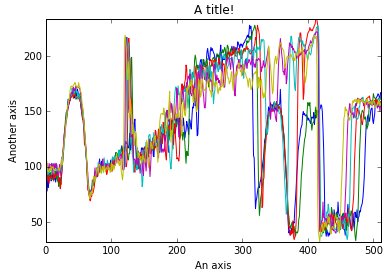Customising the figure by setting the matplotlib axes properties.

A matplotlib ax and fig object can also be specified, which can be used to put several subplots in the same figure. This will only work for “cascade” and “overlap” styles:

```>>> import scipy.misc
>>> fig, axarr = plt.subplots(1,2)
>>> s1 = hs.signals.Signal1D(scipy.misc.lena()[100:160:10])
>>> s2 = hs.signals.Signal1D(scipy.misc.lena()[200:260:10])
>>> fig.canvas.draw()
```

#### Plotting several signals¶

`plot_signals()` is used to plot several signals at the same time. By default the navigation position of the signals will be synced, and the signals must have the same dimensions. To plot two spectra at the same time:

```>>> import scipy.misc
>>> s1 = hs.signals.Signal1D(scipy.misc.face()).as_spectrum(0)[:,:3]
>>> s2 = s1.deepcopy()*-1
>>> hs.plot.plot_signals([s1, s2])
```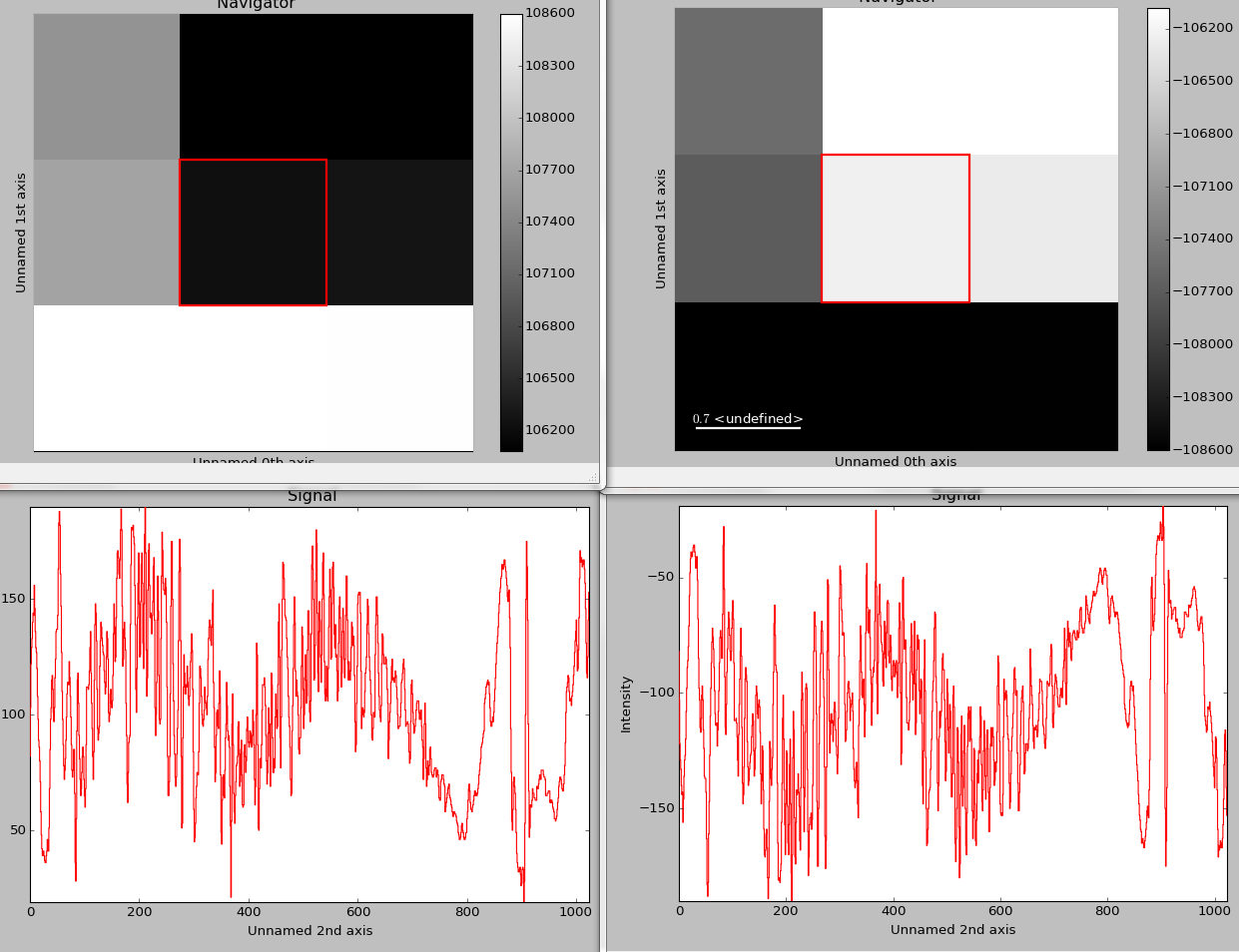The `plot_signals()` plots several signals with optinal syncronised navigation.

The navigator can be specified by using the navigator argument, where the different options are “auto”, None, “spectrum”, “slider” or Signal. For more details about the different navigators, see navigator_options. To specify the navigator:

```>>> import scipy.misc
>>> s1 = hs.signals.Signal1D(scipy.misc.face()).as_spectrum(0)[:,:3]
>>> s2 = s1.deepcopy()*-1
>>> hs.plot.plot_signals([s1, s2], navigator="slider")
```

Navigators can also be set differently for different plots using the navigator_list argument. Where the navigator_list be the same length as the number of signals plotted, and only contain valid navigator options. For example:

```>>> import scipy.misc
>>> s1 = hs.signals.Signal1D(scipy.misc.face()).as_spectrum(0)[:,:3]
>>> s2 = s1.deepcopy()*-1
>>> s3 = hs.signals.Signal1D(np.linspace(0,9,9).reshape([3,3]))
>>> hs.plot.plot_signals([s1, s2], navigator_list=["slider", s3])
```

Several signals can also be plotted without syncing the navigation by using sync=False. The navigator_list can still be used to specify a navigator for each plot:

```>>> import scipy.misc
>>> s1 = hs.signals.Signal1D(scipy.misc.face()).as_spectrum(0)[:,:3]
>>> s2 = s1.deepcopy()*-1
>>> hs.plot.plot_signals([s1, s2], sync=False, navigator_list=["slider", "slider"])
```

## Markers¶

New in version 0.8.

Hyperspy provides an easy access to the main marker of matplotlib. The markers can be used in a static way

```>>> import scipy.misc
>>> im = hs.signals.Signal2D(scipy.misc.lena())
>>> m = hs.plot.markers.rectangle(x1=150, y1=100, x2=400, y2=400, color='red')
```

By providing an array of positions, the marker can also change position when navigating the signal. In the following example, the local maxima are displayed for each R, G and B channel of a colour image.

```>>> from skimage.feature import peak_local_max
>>> import scipy.misc
>>> ims = hs.signals.Signal(scipy.misc.face()).as_image([0,1])
>>> index = array([peak_local_max(im.data, min_distance=100, num_peaks=4)
>>>                for im in ims])
>>> for i in range(4):
>>>     m = hs.plot.markers.point(x=index[:, i, 1],
>>>                                  y=index[:, i, 0], color='red')
```

The markers can be added to the navigator as well. In the following example, each slice of a 2D spectrum is tagged with a text marker on the signal plot. Each slice is indicated with the same text on the navigator.

```>>> s = hs.signals.Signal1D(np.arange(100).reshape([10,10]))
>>> s.plot(navigator='spectrum')
>>> for i in range(s.axes_manager.shape):
>>>     m = hs.plot.markers.text(y=s.sum(-1).data[i]+5,
>>>                                 x=i, text='abcdefghij'[i])